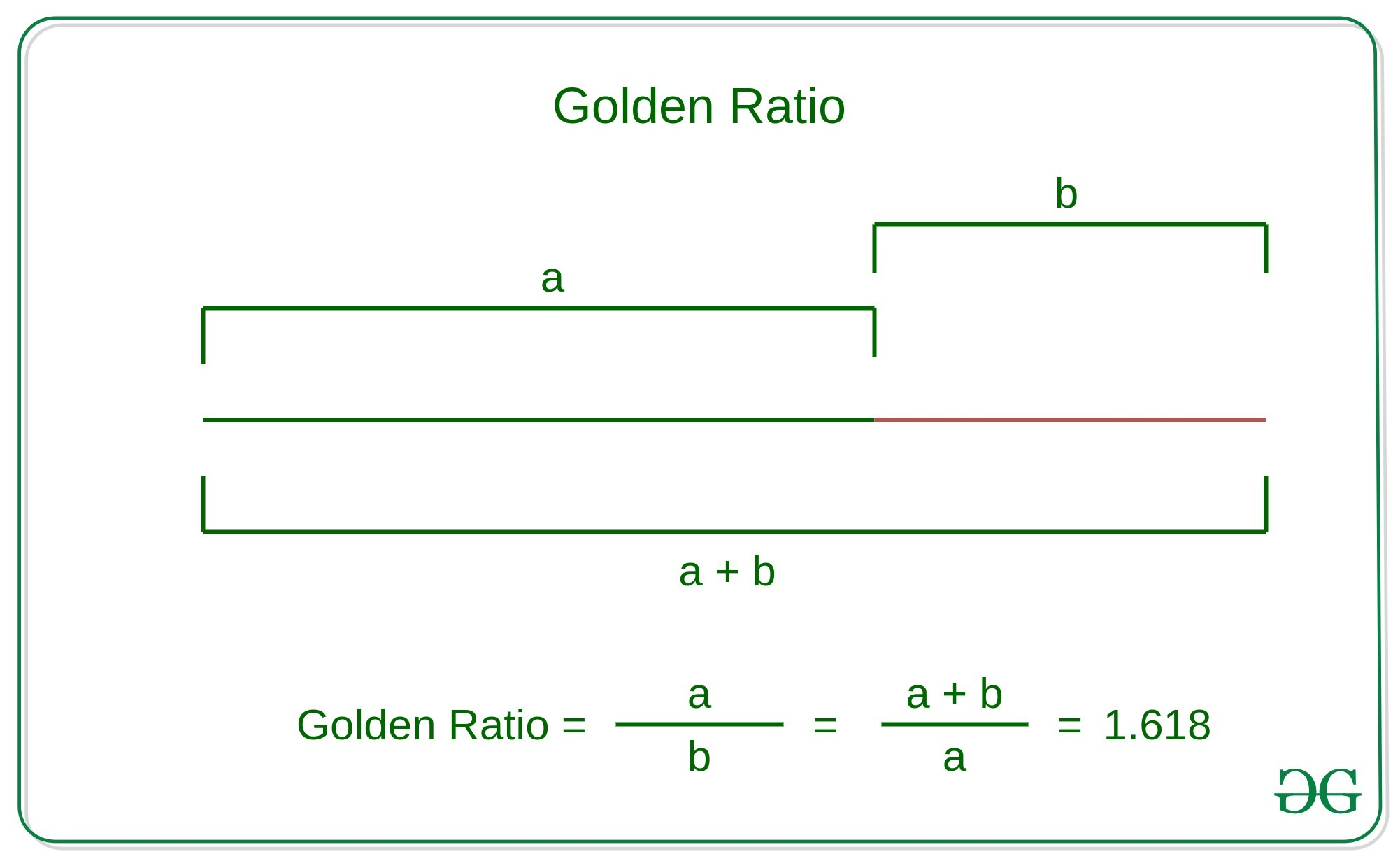Related Articles
Check whether two numbers are in golden ratio
• Difficulty Level : Expert
• Last Updated : 18 Dec, 2020

Given two numbers A and B, the task is to check that A and B are in the golden ratio.
Golden Ratio: Two numbers are said to be in the golden ratio if their ratio is the same as the ratio of the sum of the two numbers to the larger number. Here a > b > 0, Below is the geometric representation of the Golden ratio:Examples:

Input: A = 1, B = 0.618
Output: Yes
Explanation:
These two numbers together forms Golden ratioInput: A = 61.77, B = 38.22

Output Yes

Explanation:

These two numbers together forms Golden ratioApproach: The idea is to find two ratios and check that this ratio is equal to the Golden ratio. That is 1.618.

// Here A denotes the larger numberBelow is the implementation of the above approach:

## C++

 // C++ implementation to check // whether two numbers are in // golden ratio with each other#include using namespace std; // Function to check that two // numbers are in golden ratiobool checkGoldenRatio(float a,                      float b){  // Swapping the numbers such   // that A contains the maximum  // number between these numbers  if(a <= b)  {    float temp = a;    a = b;    b = temp;  }   // First Ratio  std::stringstream ratio1;  ratio1 << std :: fixed <<            std :: setprecision(3) <<            (a / b);   // Second Ratio  std::stringstream ratio2;  ratio2 << std :: fixed <<            std :: setprecision(3) <<            (a + b) / a;   // Condition to check that two  // numbers are in golden ratio  if((ratio1.str() == ratio2.str()) &&      ratio1.str() == "1.618")  {    cout << "Yes" << endl;    return true;  }  else  {    cout << "No" << endl;    return false;  }}  // Driver codeint main(){  float a = 0.618;  float b = 1;   // Function Call  checkGoldenRatio(a, b);   return 0;} // This code is contributed by divyeshrabadiya07

## Java

 // Java implementation to check // whether two numbers are in // golden ratio with each otherclass GFG{     // Function to check that two // numbers are in golden ratiopublic static Boolean checkGoldenRatio(float a,                                       float b){         // Swapping the numbers such     // that A contains the maximum    // number between these numbers    if (a <= b)    {        float temp = a;        a = b;        b = temp;    }         // First Ratio    String ratio1 = String.format("%.3f", a / b);         // Second Ratio    String ratio2 = String.format("%.3f", (a + b) / a);         // Condition to check that two    // numbers are in golden ratio    if (ratio1.equals(ratio2) &&        ratio1.equals("1.618"))    {        System.out.println("Yes");        return true;    }    else    {        System.out.println("No");          return false;    }} // Driver codepublic static void main(String []args){    float a = (float)0.618;    float b = 1;         // Function Call    checkGoldenRatio(a, b);}} // This code is contributed by rag2127

## Python3

 # Python3 implementation to check# whether two numbers are in# golden ratio with each other # Function to check that two# numbers are in golden ratiodef checkGoldenRatio(a, b):         # Swapping the numbers such    # that A contains the maximum    # number between these numbers    a, b = max(a, b), min(a, b)         # First Ratio    ratio1 = round(a/b, 3)         # Second Ratio    ratio2 = round((a+b)/a, 3)    # Condition to check that two    # numbers are in golden ratio    if ratio1 == ratio2 and\       ratio1 == 1.618:        print("Yes")        return True    else:        print("No")        return False         # Driver Codeif __name__ == "__main__":    a = 0.618    b = 1         # Function Call    checkGoldenRatio(a, b)

## C#

 // C# implementation to check // whether two numbers are in // golden ratio with each otherusing System;using System.Collections.Generic;class GFG {         // Function to check that two     // numbers are in golden ratio    static bool checkGoldenRatio(float a,                          float b)    {      // Swapping the numbers such       // that A contains the maximum      // number between these numbers      if(a <= b)      {        float temp = a;        a = b;        b = temp;      }            // First Ratio      string ratio1 = String.Format("{0:0.000}", a / b);            // Second Ratio      string ratio2 = String.Format("{0:0.000}", (a + b) / a);       // Condition to check that two      // numbers are in golden ratio      if(ratio1 == ratio2 && ratio1 == "1.618")      {        Console.WriteLine("Yes");        return true;      }      else      {        Console.WriteLine("No");        return false;      }    }     // Driver code   static void Main() {      float a = (float)0.618;      float b = 1;            // Function Call      checkGoldenRatio(a, b);  }} // This code is contributed by divyesh072019
Output:
Yes

References: https://en.wikipedia.org/wiki/Golden_ratio

Attention reader! Don’t stop learning now. Get hold of all the important DSA concepts with the DSA Self Paced Course at a student-friendly price and become industry ready.

My Personal Notes arrow_drop_up